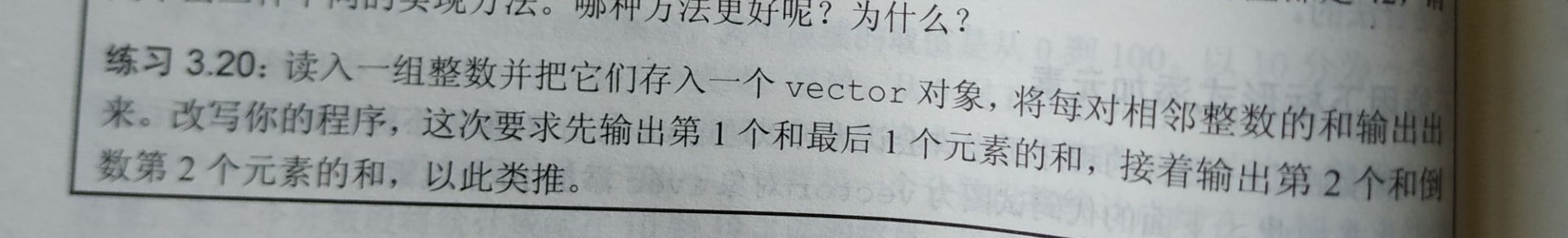c++ vector容器中push_back函数输入一组数据时为什么跳不出输入的while循环？``````#include<iostream>
#include<vector>
using namespace::std;
int main()
{
vector<int>num;
int word, sum=0;
while (cin >> word)
{
num.push_back(word);
}
for (unsigned int i = 0; i < num.size() - 1; i++)
{
sum = num[i] + num[i + 1];
}
cout << sum << endl;
}
``````

2个回答